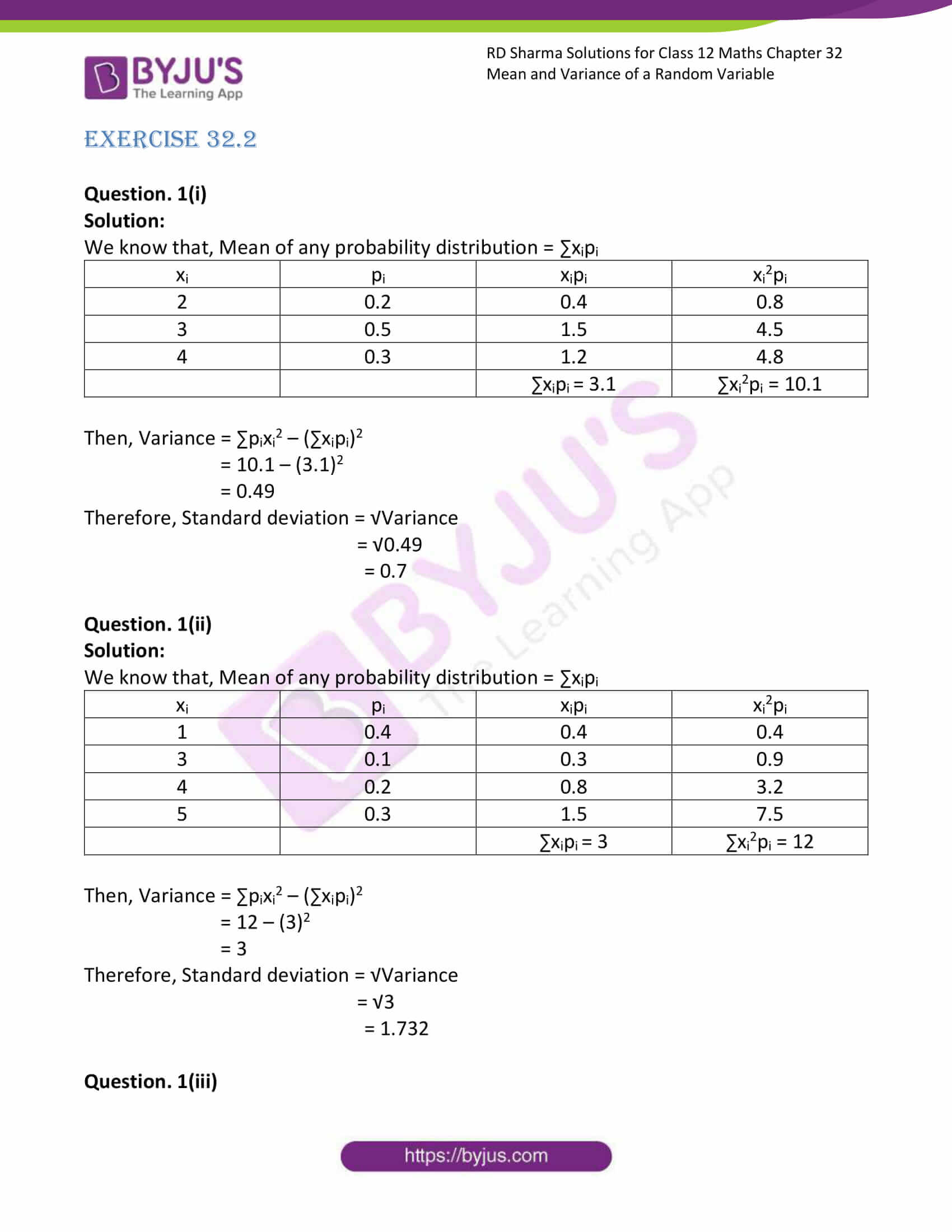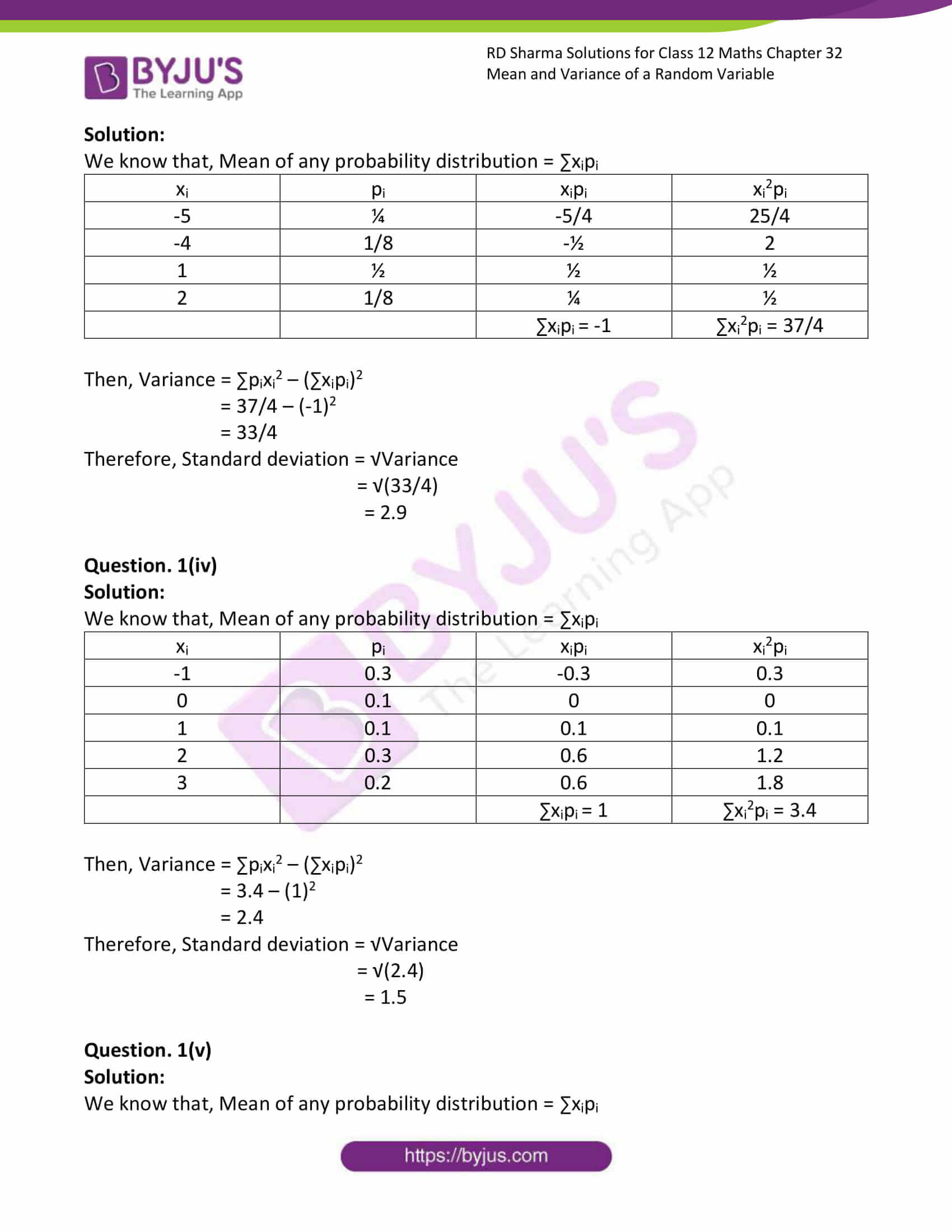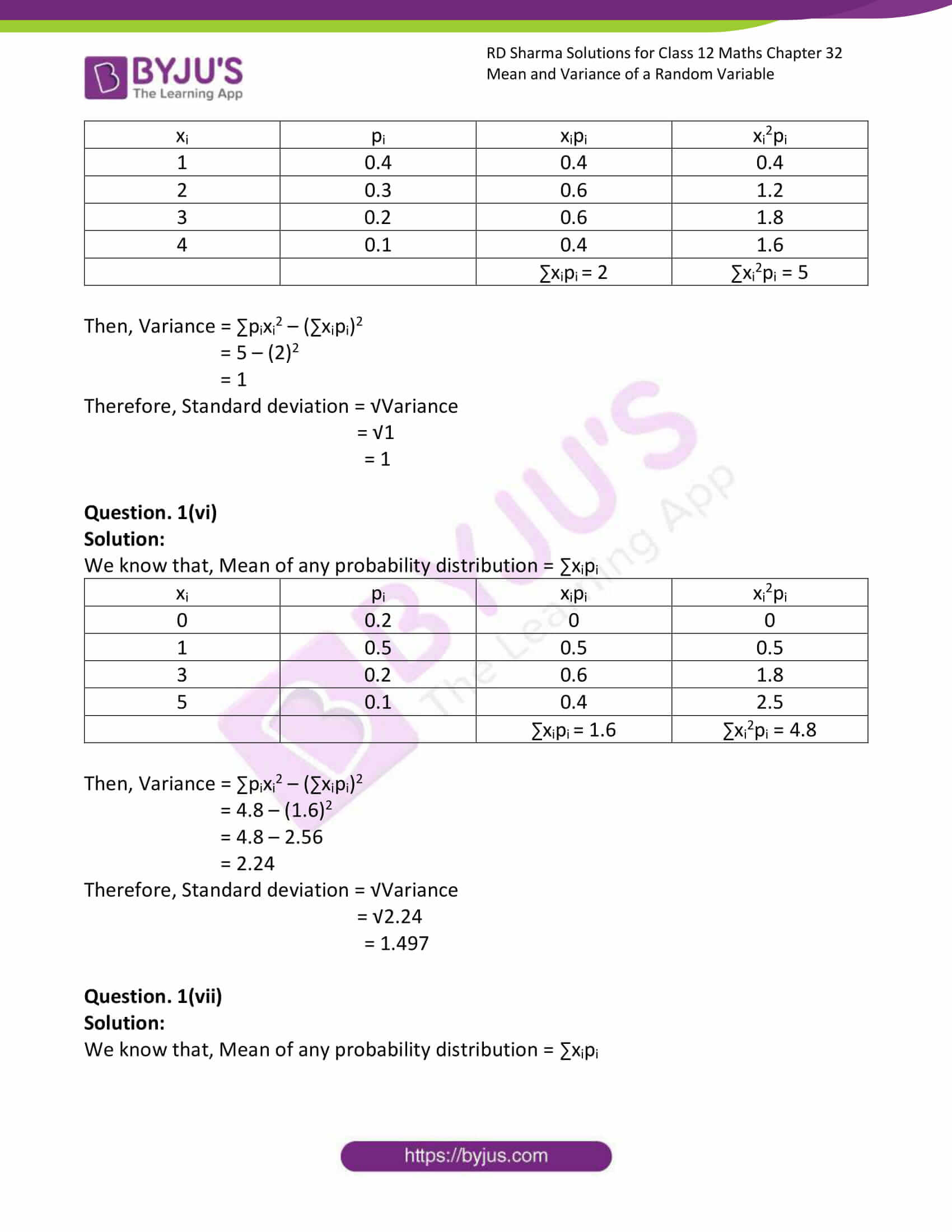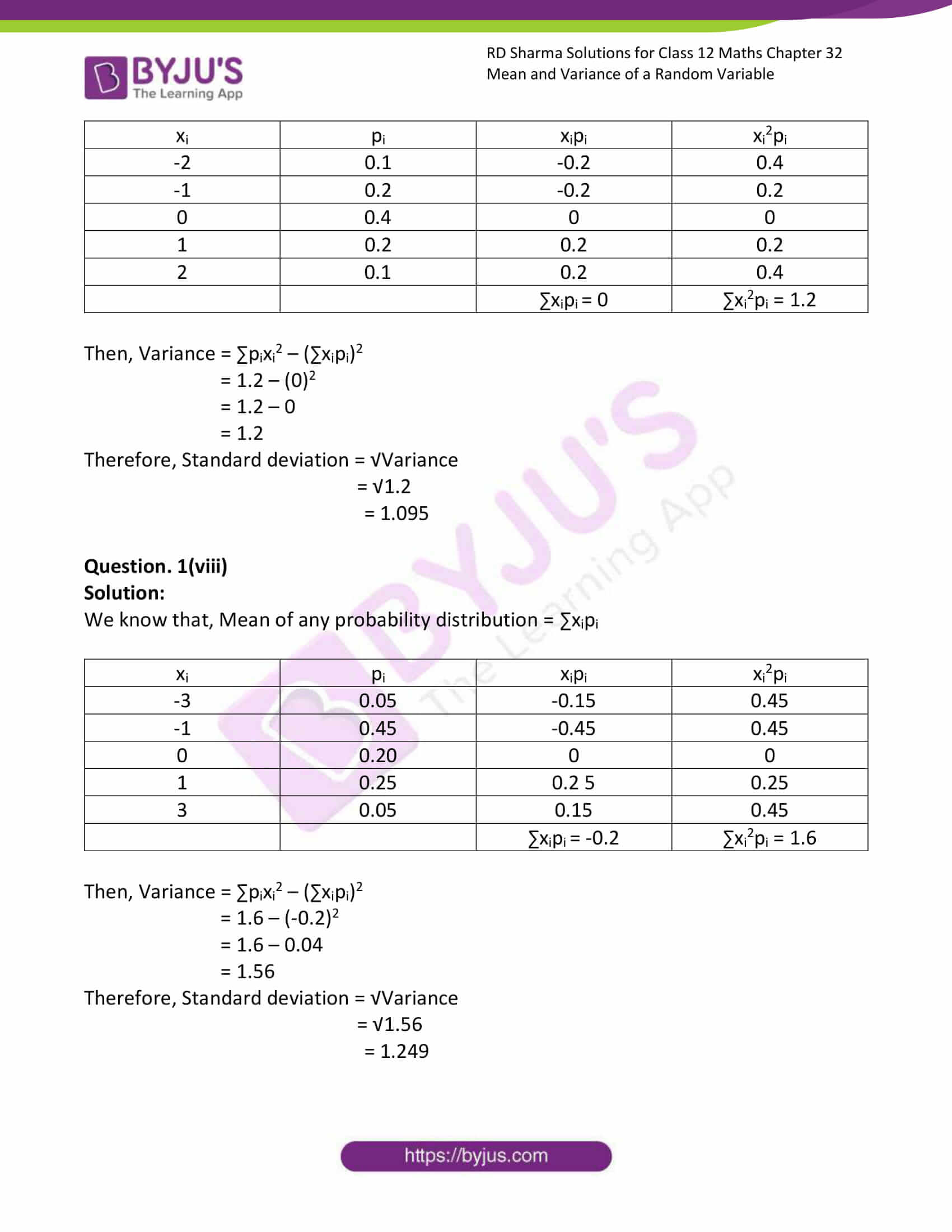# RD Sharma Solutions Class 12 Mean And Variance Of A Random Variable Exercise 32.2

RD Sharma Solutions for Class 12 Maths Exercise 32.1 Chapter 32 Mean and Variance of a Random Variable are available here. Our expert tutors formulate the exercises to assist you with your exam preparation to attain good marks in Maths. Students who wish to score good marks in Maths practise RD Sharma Solutions for Class 12 Maths Chapter 32.

## Download PDF of Rd Sharma Solution for Class 12 Maths Chapter 32 Exercise 2### Access Answers for Rd Sharma Solution Class 12 Maths Chapter 32 Exercise 2

EXERCISE 32.2

Question. 1(i)

Solution:

We know that, Mean of any probability distribution = ∑xipi

 xi pi xipi xi2pi 2 0.2 0.4 0.8 3 0.5 1.5 4.5 4 0.3 1.2 4.8 ∑xipi = 3.1 ∑xi2pi = 10.1

Then, Variance = ∑pixi2 – (∑xipi)2

= 10.1 – (3.1)2

= 0.49

Therefore, Standard deviation = √Variance

= √0.49

= 0.7

Question. 1(ii)

Solution:

We know that, Mean of any probability distribution = ∑xipi

 xi pi xipi xi2pi 1 0.4 0.4 0.4 3 0.1 0.3 0.9 4 0.2 0.8 3.2 5 0.3 1.5 7.5 ∑xipi = 3 ∑xi2pi = 12

Then, Variance = ∑pixi2 – (∑xipi)2

= 12 – (3)2

= 3

Therefore, Standard deviation = √Variance

= √3

= 1.732

Question. 1(iii)

Solution:

We know that, Mean of any probability distribution = ∑xipi

 xi pi xipi xi2pi -5 ¼ -5/4 25/4 -4 1/8 -½ 2 1 ½ ½ ½ 2 1/8 ¼ ½ ∑xipi = -1 ∑xi2pi = 37/4

Then, Variance = ∑pixi2 – (∑xipi)2

= 37/4 – (-1)2

= 33/4

Therefore, Standard deviation = √Variance

= √(33/4)

= 2.9

Question. 1(iv)

Solution:

We know that, Mean of any probability distribution = ∑xipi

 xi pi xipi xi2pi -1 0.3 -0.3 0.3 0 0.1 0 0 1 0.1 0.1 0.1 2 0.3 0.6 1.2 3 0.2 0.6 1.8 ∑xipi = 1 ∑xi2pi = 3.4

Then, Variance = ∑pixi2 – (∑xipi)2

= 3.4 – (1)2

= 2.4

Therefore, Standard deviation = √Variance

= √(2.4)

= 1.5

Question. 1(v)

Solution:

We know that, Mean of any probability distribution = ∑xipi

 xi pi xipi xi2pi 1 0.4 0.4 0.4 2 0.3 0.6 1.2 3 0.2 0.6 1.8 4 0.1 0.4 1.6 ∑xipi = 2 ∑xi2pi = 5

Then, Variance = ∑pixi2 – (∑xipi)2

= 5 – (2)2

= 1

Therefore, Standard deviation = √Variance

= √1

= 1

Question. 1(vi)

Solution:

We know that, Mean of any probability distribution = ∑xipi

 xi pi xipi xi2pi 0 0.2 0 0 1 0.5 0.5 0.5 3 0.2 0.6 1.8 5 0.1 0.4 2.5 ∑xipi = 1.6 ∑xi2pi = 4.8

Then, Variance = ∑pixi2 – (∑xipi)2

= 4.8 – (1.6)2

= 4.8 – 2.56

= 2.24

Therefore, Standard deviation = √Variance

= √2.24

= 1.497

Question. 1(vii)

Solution:

We know that, Mean of any probability distribution = ∑xipi

 xi pi xipi xi2pi -2 0.1 -0.2 0.4 -1 0.2 -0.2 0.2 0 0.4 0 0 1 0.2 0.2 0.2 2 0.1 0.2 0.4 ∑xipi = 0 ∑xi2pi = 1.2

Then, Variance = ∑pixi2 – (∑xipi)2

= 1.2 – (0)2

= 1.2 – 0

= 1.2

Therefore, Standard deviation = √Variance

= √1.2

= 1.095

Question. 1(viii)

Solution:

We know that, Mean of any probability distribution = ∑xipi

 xi pi xipi xi2pi -3 0.05 -0.15 0.45 -1 0.45 -0.45 0.45 0 0.20 0 0 1 0.25 0.2 5 0.25 3 0.05 0.15 0.45 ∑xipi = -0.2 ∑xi2pi = 1.6

Then, Variance = ∑pixi2 – (∑xipi)2

= 1.6 – (-0.2)2

= 1.6 – 0.04

= 1.56

Therefore, Standard deviation = √Variance

= √1.56

= 1.249